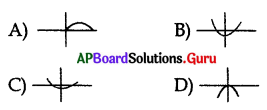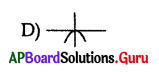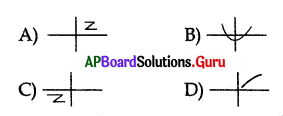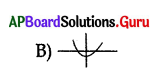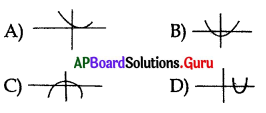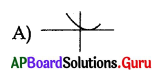Practice the AP 10th Class Maths Bits with Answers Chapter 3 Polynomials on a regular basis so that you can attempt exams with utmost confidence.

## AP State Syllabus 10th Class Maths Bits 3rd Lesson Polynomials with Answers

Question 1.
Zero of the polynomial (3x – 1) is ………………
A) 3
B) 0
C) $$\frac{1}{3}$$
D) 1
C) $$\frac{1}{3}$$

Question 2.
The zeroes of the polynomial (shown in the graph) are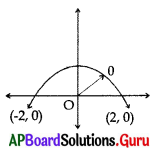A) 2, 0
B) 0,-2
C) -2, 2
D) 0, 0
C) -2, 2Question 3.
The remainder of 3x3 – 2x2 + x + 2 when divided by 3x + 1 is
A) $$\frac{4}{3}$$
B) $$\frac{3}{4}$$
C) –$$\frac{4}{3}$$
D) None
A) $$\frac{4}{3}$$

Question 4.
If α, β, are the roots of 5x2 + 5x + 6 = 0, then (1 + α) (1 + β) =
A) ) –$$\frac{4}{3}$$
B) ) $$\frac{3}{5}$$
C) ) $$\frac{6}{5}$$
D) ) –$$\frac{6}{5}$$
C) ) $$\frac{6}{5}$$

Question 5.
If two zeroes of the polynomial x3 – 5x2 + 6x are 2 and 3, then the third zero is
A) 0
B) 5
C) 6
D) 1
A) 0

Question 6.
If ‘4’ is one of the zeroes of p(x) = x2 + kx – 8, then the value of k = ………………
A) 1
B) -1
C) 2
D) -2
D) -2

Question 7.
If the roots of x2 + 6x + 5 = 0 are α and β, then α + β = ………………..
A) 5
B) -6
C) 6
D) -1
B) -6

Question 8.
If α, β are the zeroes of x2 + x + 1, then $$\frac{1}{\alpha}+\frac{1}{\beta}$$ = ……………………
A) 1
B) -1
C) 2
D) -2
B) -1

Question 9.
The degree of the polynomial
√2 X2– 3X + 1 = …………………..
A) √2
B) 3
C) 1
D) 2
D) 2Question 10.
If one zero of the polynomial f (x) = 5x2 + 13x + k is reciprocal of the , other, then the value of k = …………………..
A) 13
B) 5
C) -5
D) – $$\frac{13}{5}$$
B) 5

Question 11.
A quadratic polynomial, the sum of whose zeroes is zero and one zero is 4, is ………………..
A) x2 – 16
B) x2 + 16
C) x2 – 4
D) x2 + 4
A) x2 – 16

Question 12.
Sum of zeroes of a polynomial x3 – 2x2 + 3x – 4 is ……………….
A) -2
B) 2
C) 1
D) 4
B) 2

Question 13.
The number of zeroes of the polynomial whose graph is given below: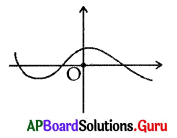A) 0
B) 1
C) 2
D) 3
D) 3

Question 14.
The graph represented by y = x is …………………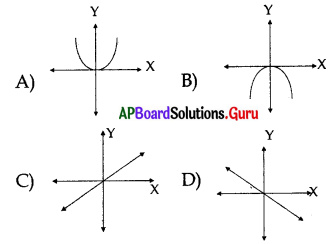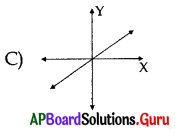Question 15.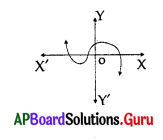Number of zeroes can be identified by the adjacent figure.
A) 0
B) 1
C) 2
D) 3
D) 3

Question 16.
If α and β are the zeroes of the polynomial p(x) = 3x2 – x – 4, then αβ = ………………..
A) $$\frac{1}{3}$$
B) –$$\frac{1}{3}$$
C) $$\frac{4}{3}$$
D) –$$\frac{4}{3}$$
D) –$$\frac{4}{3}$$Question 17.
If p(x) = 5x7 – 6x5 + 7x – 6, then the degree of p (x) is …………….
A) 0
B) 1
C) 5
D) 7
D) 7

Question 18.
A factor of x3 – 3x2 + x + 1 is
A) x + 1
B) 2x – 1
C) 2x + 1
D) x – 1
D) x – 1

Question 19.
The length of a rectangular dining hall is twice of its breadth. If x represents the breadth of the hall and its area is 5 sq. units, then the polynomial equation which represents the situation is
A) 5x2 – 2 = 0
B) 2x2 – 5 = 0
C) x2 – 25 = 0
D) None
B) 2x2 – 5 = 0

Question 20.
The sum of the zeros of the polynomial p(x) = x2 + 7x + 10 is
A) 7
B) -7
C) 10
D) -10
B) -7

Question 21.
If p(x) = 2x2 + 3x – 5, then p(2) =
A) 2
B) 9
C) 0
D) -5
B) 9

Question 22.
If p (x) = 3x – k and p (1) = 0 then k =
A) 3
B) -3
C) -1
D) +1
A) 3

Question 23.
The value of (x – √3)(x + √3) = ………………..
A) x2 + 3
B) x2 – 3
C) x + 9
D) x – 9
B) x2 – 3Question 24.
If α and β are the two zeroes of a polynomial x2 – 5x + 6 then the value of $$\frac{1}{a^{2}}+\frac{1}{\beta^{2}}$$ is ……………….
A) $$\frac{13}{36}$$
B) $$\frac{17}{36}$$
C) $$\frac{19}{36}$$
D) $$\frac{23}{36}$$
A) $$\frac{13}{36}$$

Question 25.
If α, β are the two zeroes of the polynomial f(x) = x2 – p (x +1) – c, then (α + 1)(β + 1) = …………………
A) c – 1
B) 1 – c
C) c
D) 1 + c
B) 1 – c

Question 26.
If α and β are the roots of ax2 + bx + c = 0, then α3 + β3 =
A) $$\frac{3 a b c-b^{3}}{a^{3}}$$
B) $$\frac{3 a b c-b^{3}}{c^{3}}$$
C) $$\frac{b^{2}-3 a b c}{a^{3}}$$
D) $$\frac{b^{2}-3 a b c}{c^{3}}$$
A) $$\frac{3 a b c-b^{3}}{a^{3}}$$

Question 27.
The equation whose roots are obtained by adding 1 to those of 2x2 + 3x + 5 = 0 is
A) 2x2 – x – 4 = 0
B) 2x2 + x – 4 = 0
C) 2x22 – x + 4 = 0
D) None
C) 2x22 – x + 4 = 0

Question 28.
If x + 1, x + 2 are two factors of x3 + 3x2 -2αx + β, then values of α + β is ……………………
A) – 1
B) 1
C) -2
D) 2
A) – 1

Question 29.
If α, β are zeroes of the polynomial
ax2 + bx + c, then the value of $$\frac{1}{\alpha}+\frac{1}{\beta}$$ is…………………
A) $$\frac{-b}{a c}$$
B) b = ac
C) $$\frac{-b}{c}$$
D) $$-\sqrt{\frac{b}{a c}}$$
C) $$\frac{-b}{c}$$

Question 30.
The value of ‘K’ for which 2x4 + 3x3 + 2Kx2 + 3x + 6 is exactly divisible by x + 2?
A) 1
B) -1
C) 2
D) -2
B) -1Question 31.
The degree of the polynomial 5x7 – 6x5 + 7x – 6 is
A) 4
B) 5
C) 6
D) 7
D) 7

Question 32.
The zero of the linear polynomial px + q is ………………..
A) $$-\frac{q}{p}$$
B) $$\frac{\mathrm{p}}{\mathrm{q}}$$
C) $$-\frac{p}{q}$$
D) q
A) $$-\frac{q}{p}$$

Question 33.
The quadratic polynomial, whose zeroes are 2 and 3, is
A) x2 – 5x – 6
B) x2 + 5x – 6
C) x2 – 5x + 6
D) x2 + 5x + 6
C) x2 – 5x + 6

Question 34.
Which of the following is a polynomial with only one zero ?
A) p(x) = 2x2 – 3x + 4
B) p(x) = x2 – 2x + 1
C) p(x) = 2x + 3
D) p(x) = 5
C) p(x) = 2x + 3

Question 35.
Observe the given rectangular figure, then its area in polynomial function is …………………..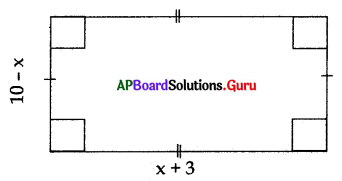A) A(x) = x2 + 7x + 30
B) A(x) = – x2 + 7x + 30
C) A(x) = x2 – 7x + 30
D) A (x) = -x2 – 7x + 30
B) A(x) = – x2 + 7x + 30

Question 36.
For the expression f(x) = x3 + ax2 + bx + c if f(1) = f(2) = 0 and f(4) = f(0). Find the values of a, b, c.
A) a = -8, b = -20, c = 12
B) a = 9, b = -20, c = -12
C) a = -9, b = 20, c = -12
D) a = -8, b =. 20, c = -12
C) a = -9, b = 20, c = -12

Question 37.
If (x4 + ax3 – 7x2 – 8x + b) is completely divisible by (x2 + 5x + 6). Then the values of a and b are ……………………..
A) a = 2, b = 8
B) a = -2, b = 6
C) a = 2, b = 12
D) a = 2, b = 14
C) a = 2, b = 12Question 38.
x3 + 2X2 + ax + b is exactly divisible by (x2 – 1). The values of a and b is ………………
A) a = -1, b = 2
B) a = 1, b = -2
C) a = 1, b = 2
D) a = -1, b = -2
D) a = -1, b = -2

Question 39.
Find the remainder when x4 + 15x3 + 6x2 – 12x + 3 is divided by x + 2 ? ………………….
A) -53
B) 53
C) -52
D) 52
A) -53

Question 40.
If x2 + x -1 is a factor of x4 + px3 + qx2 – 1 then the values of p and q can be …………………
A) 2, 1
B) -2, -1
C) -1,-2
D) 1, -2
A) 2, 1

Question 41.
If the zeroes of the rational expression (ax + b) (3x + 2) are $$\frac{-2}{3}$$ and $$\frac{1}{2}$$ then a + b = …………………..
A) -1
B) 0
C) -b
D) 1
C) -b

Question 42.
Simplify $$\frac{x+1}{x-1}+\frac{x-1}{x+1}-\frac{2 x^{2}-2}{x^{2}+1}$$
A) $$\frac{4 x^{4}+2}{x^{4}-1}$$
B) $$\frac{8 x^{2}}{x^{4}-1}$$
C) $$\frac{4 x^{2}}{x^{4}-1}$$
D) 1
B) $$\frac{8 x^{2}}{x^{4}-1}$$

Question 43.
Find f(4),f(-5),f (3.2) if f (x) = 6.2x2 – 4x3 + 4.28 ………………
A) f(4) = -152.53, f(-5) = 659.28, f(3.2) = 63.304
B) f(4) = -152.52, f(-5) = -659.28, f(3.2) = 63.304
C) f(4) = -152.52, f(-5) = 659.28, f(3.2) = -63.304
D) f(4) = -152.52, f(-5) = 659.27, f(3.2) = -63.304
C) f(4) = -152.52, f(-5) = 659.28, f(3.2) = -63.304

Question 44.
The product of x3 + 2x2 – 3x + 4 and 2x2 – 5x + 1 is …………………
A) 2x5 – x4 – 15x3 + 25x2 – 23x + 4
B) 2x5 – x4 – 15x3 + 25x2 – 23x – 4
C) 2x5 – x4 – 15x3 – 25x2 – 23x – 4
D) 2x5 – x4 – 15x3 – 23x – 4
A) 2x5 – x4 – 15x3 + 25x2 – 23x + 4Question 45.
If A = $$\frac{x-1}{x+1}$$ then 2A – $$\frac{1}{2 A}$$ = ……………….
A) $$\frac{3 x^{2}-10 x-3}{2\left(x^{2}-1\right)}$$
B) $$\frac{3 x^{2}-10 x+3}{2\left(x^{2}-1\right)}$$
C) $$\frac{3 x^{2}+10 x+3}{2\left(x^{2}-1\right)}$$
D) $$\frac{3 x^{2}-10 x+1}{x^{2}-1}$$
B) $$\frac{3 x^{2}-10 x+3}{2\left(x^{2}-1\right)}$$

Question 46.
If P = $$\frac{x+1}{x-1}$$ and Q = $$\frac{x-1}{x+1}$$ then P2 + Q2 – 2PQ = …………………..
A) $$\frac{4 x^{4}+8 x^{2}+4}{x^{4}-2 x+1}$$
B) $$\frac{4 x^{2}}{x^{4}+2 x^{2}+1}$$
C) $$\frac{16 x^{2}}{x^{4}-2 x^{2}+1}$$
D) $$\frac{8 x^{2}}{x^{4}-2 x^{2}+1}$$
C) $$\frac{16 x^{2}}{x^{4}-2 x^{2}+1}$$

Question 47.
The rational expression A = $$\left(\frac{x+1}{x-1}-\frac{x-1}{x+1}-\frac{4 x}{x^{2}+1}\right)$$ is multiplied with the additive inverse of B = $$\frac{1-x^{4}}{4 x}$$ to get C, then C = ……………..
A) 2
B) $$\frac{2 x}{x^{4}-1}$$
C) $$\frac{32 x^{2}}{x^{4}-1}$$
D) 1
A) 2

Question 48.
The product of additive inverse of $$\frac{x^{2}-1}{2 x}$$ and $$\frac{x^{2}-4}{3-x}$$ is …………………….
A) x2 + x – 6
B) x2 – x – 6
C) x2 – 5x + 6
D) x2 + 5x + 6
A) x2 + x – 6

Question 49.
If (x – 1) and (x + 3) are the factors of x3 + 3x2 – x – 3 then the other factor is …………………
A) x + 1
B) x – 3
C) x + 2
D) x – 1
A) x + 1

Question 50.
A rational expression whose numerator is a quadratic polynomial with zeroes, 1 and 2 and whose denominator is monomial with zero 3 is ………………….
A) $$\frac{x^{2}+x-2}{x+3}$$
B) $$\frac{x^{2}-x-2}{x+3}$$
C) $$\frac{x^{2}+x-2}{x-3}$$
D) $$\frac{x^{2}-x-2}{x-3}$$
D) $$\frac{x^{2}-x-2}{x-3}$$

Question 51.
Simplify $$\frac{\left(a^{2}-b^{2}\right)^{3}+\left(b^{2}-c^{2}\right)^{3}+\left(c^{2}-a^{2}\right)^{3}}{(a-b)^{3}+(b-c)^{3}+(c-a)^{3}}$$
A) 1
B) 3(a + b) (b + c) (c + a)
C) (a + b) (b + c) (c + a)
D) 2(a + b) (b + c) (c + a)
C) (a + b) (b + c) (c + a)Question 52.
Find “a” and “b” in order that x3 – 6x2 + ax + b may be exactly divisible by x2 – 3x + 2.
A) a = 11, b = -5
B) a = 11, b = 6
C) a = 11, b = -6
D) a = -11, b = -5
B) a = 11, b = 6

Question 53.
If g(x) = x6 + 3x4 – 24x2 + 3 find g(1), g(2) and g(3).
A) g(1) = -12, g(2) = 19, g(3) = 759
B) g(1) = 12, g(2) = 19, g(3) = 758
C) g(1) = -12, g(2) = -19, g(3) = -759
D) g(1) = -12, g(2) = -19, g(3) = 759
A) g(1) = -12, g(2) = 19, g(3) = 759

Question 54.
If the zeroes of the rational expression (3x + 2a) (2x + 1) are $$\frac{-1}{2}$$ and $$\frac{\mathbf{b}}{3}$$ then the value of a is ……………………..
A) $$\frac{-b}{3}$$
B) $$\frac{-b}{2}$$
C) $$\frac{-2 b}{3}$$
D) $$\frac{2 b}{3}$$
B) $$\frac{-b}{2}$$

Question 55.
If ‘a’ and ‘b’ are unequal and x2 + ax + b and x2 + bx + a have a common factor, then a + b = ……………………
A) -1
B) 1
C) 0
D) 2
A) -1

Question 56.
Find the quadratic polynomial in x, which when divided by (x – 1) (x – 2) and (x – 3) leaves remainders of 11, 22 and 37 …………………….
A) 2x2 + 5x + 4
B) 2x2 – 5x – 3
C) 3x2 – 5x + 4
D) 2x2 – 5x + 4
A) 2x2 + 5x + 4

Question 57.
The sum of $$\left(\frac{x-1}{x+1}\right)$$ and its reciprocal is ……………………
A) $$\frac{x^{2}+1}{x^{2}-1}$$
B) $$\frac{2\left(x^{2}+1\right)}{x^{2}-1}$$
C) $$\frac{x^{2}-1}{x^{2}+1}$$
D) $$\frac{2\left(x^{2}-1\right)}{x^{2}+1}$$
B) $$\frac{2\left(x^{2}+1\right)}{x^{2}-1}$$

Question 58.
The value of 6a + 11b if x3 – 6x2 + ax + b is exactly divisible by (x2 – 3x + 2) is ………………………
A) 0
B) 66
C) 132
D) 1
A) 0Question 59.
If 4x4 -(a – 1) x3 + ax2 – 6x – 1 is divisible by 2x – 1, the value of a …………………..
A) 28
B) 29
C) 27
D) 26
B) 29

Question 60.
If f(x) = x2 + 5x + p and g(x) = x2 + 3x + q have a common factor then (p – q)2 = ………………….
A) 2(3p – 5q)
B) 5p – 3q
C) 3p – 5q
D) 2(5p – 3q)
A) 2(3p – 5q)

Question 61.
If (x – 4) is the HCF of p(x) = x2 – nx -12 and q(x) =x2 – mx – 8 then the simplest form of $$\frac{p(x)}{q(x)}$$ is …………………
A) $$\frac{x+2}{x+3}$$
B) $$\frac{x+3}{x-2}$$
C) $$\frac{x+3}{x+2}$$
D) $$\frac{x-2}{x-3}$$
B) $$\frac{x+3}{x-2}$$

Question 62.
Simplify $$\frac{x^{2}-(y-2 z)^{2}}{x-y+2 z}$$ + $$\frac{y^{2}-(2 x-z)^{2}}{y+2 x-z}$$ + $$\frac{z^{2}-(x-2 y)^{2}}{z-x+2 y}$$ is ……………………
A) 1
B) x + y + z
C) 0
D) x – y – z
C) 0

Question 63.
If P= $$\frac{1+2 x}{1-2 x}$$ and Q = $$\frac{1-2 x}{1+2 x}$$ then $$\frac{P-Q}{P+Q}$$ = ………………..
A) $$-\frac{4 x}{1+4 x^{2}}$$
B) $$\frac{1+4 x^{2}}{4 x}$$
C) $$\frac{-(1+4 x)^{2}}{4 x}$$
D) $$\frac{4 x}{1+4 x^{2}}$$
A) $$-\frac{4 x}{1+4 x^{2}}$$

Question 64.
If α, β,γ are the zeroes of 3x3 – 5x2 – 11x – 3 then α + β + γ = ……………….
A) $$\frac{-5}{3}$$
B) $$\frac{5}{3}$$
C) $$\frac{-11}{3}$$
D) 1
B) $$\frac{5}{3}$$

Question 65.
If x4 – 2x3 + 3x2 – mx + 5 is exactly divisible by (x – 3) then m = …………………
A) $$\frac{-40}{3}$$
B) $$\frac{6}{40}$$
C) -40
D) 40
D) 40Question 66.
Find the values of “a” and “b” so that (x + 2) and (x – 1) may be factors of x3 + 10x2 + ax + b.
A) a = 7,b = -18
B) a = 7, b = -17
C) a = 7, b = -15
D) a = 7, b = 17
A) a = 7,b = -18

Question 67.
Simplify $$\frac{(x+y)^{2}-z^{2}}{(x+y+z)^{2}}$$ ÷ $$\left\{\frac{(x-z)^{2}-y^{2}}{x^{2}+x y+z x} \div \frac{(x-y)^{2}-z^{2}}{x^{2}-x y-z x}\right\}$$ …………………….
A) $$\frac{1}{x+y+z}$$
B) 1
C) x + y + z
D) 2
B) 1

Question 68.
The expression 2x3 + ax2 + bx – 2 leaves a remainder 7 and 0 when divided by (2x – 3) and (x + 2). Find the values of a and b.
A) a = 3, b = -3
B) a = -3, b = -3
C) a = -3, b = 3
D) a = 3, b = 3
A) a = 3, b = -3

Question 69.
Find the value of k if the expressions
p(x) = kx3 + 4x2 + 3x – 4 and
q(x) = x3 – 4x + k leave the same remainder when divided by (x – 3)
A) k = -1
B) k = 4
C) k = 3
D) k = -2
A) k = -1

Question 70.
If a + c + e = 0 and b + d = 0 then ax4 + bx3 + cx2 + dx + e is exactly divisible by ……………………….
A) x + 1
B) x – 1
C) (x + 1) and (x – 1)
D) (x + 2) and (x – 2)
C) (x + 1) and (x – 1)

Question 71.
Simplify $$\frac{1}{1+x+x^{2}}$$ – $$\frac{1}{1-x+x^{2}}$$ + $$\frac{2 x}{1+x^{2}+x^{4}}$$ ………………
A) $$\frac{1}{1+x^{2}+x^{4}}$$
B) 0
C) $$\frac{-1}{1+x^{2}+x^{4}}$$
D) $$\frac{2 x-3}{1+x^{2}+x^{4}}$$
B) 0

Question 72.
What should be subtracted from x3 – 2x2 + 4x + 1 to get 1 ? ( )
A) x3 – 2x2 + 4x + 1
B) x3 + 2x2 + 4x + 1
C) x3 – 2x2 + 4x
D) x3 – 2x2 + 4x -1
C) x3 – 2x2 + 4xQuestion 73.
What should be subtracted from $$\frac{7 x}{x^{2}-x-12}$$ to get $$\frac{3}{x+3}$$ ?
A) $$\frac{1}{x-4}$$
B) $$\frac{2}{x-4}$$
C) $$\frac{4}{x-4}$$
D) $$\frac{5}{x+4}$$
C) $$\frac{4}{x-4}$$

Question 74.
If ax2 + 2a2x + b3 is divisible by (x + a) then …………………..
A) a2 + ab + b2 = 0
B) a = b
C) either a = b or a2 + ab + b2 = 0
D) neither a = b nor a2 + ab + b2 = 0
C) either a = b or a2 + ab + b2 = 0

Question 75.
Which of the following expressions is a rational expression ?
A) $$\frac{5 x^{2}-\sqrt{6} x+7}{x+3}$$
B) $$\frac{\sqrt{2} x^{2}-4 \sqrt{x}+5}{x-\sqrt{2}}$$
C) x3 – √3x2 + √5x – 11
D) $$\frac{x^{2}+3}{2 \sqrt{x}-1}$$
C) x3 – √3x2 + √5x – 11

Question 76.
Express x – $$\frac{1}{x}$$ as a rational expression,
A) $$\frac{x^{2}-1}{x}$$
B) $$\frac{1-x^{2}}{x}$$
C) $$\frac{1-x}{x}$$
D) $$\frac{x-1}{x}$$
A) $$\frac{x^{2}-1}{x}$$

Question 77.
Which of the following expressions is a polynomial ?
A) 3x2 -2√5x + 7
B) $$\frac{x^{3}-2 x+1}{2 x+5}$$
C) $$\frac{x^{2}-5 x+6}{x-3}$$
D) $$\frac{x^{2}-2 x+1}{2 x+7}$$
C) $$\frac{x^{2}-5 x+6}{x-3}$$

Question 78.
How much is a2 + 2ab + b2 more than a2 – 2ab + b2 ?
A) -4ab
B) 4ab
C) 6ab
D) -3ab
B) 4ab

Question 79.
Which of the following is true ?
A) The sum of two rational expressions is always a rational expression
B) $$\frac{p(x)}{q(x)}$$ is in its lowest terms if LCM [p(x), q(x)] = 1.
C) The difference of two rational expressions is always a rational expression.
D) Reciprocal of $$\frac{-2 x}{x^{2}-1}$$ is $$\frac{x^{2}-1}{2 x}$$
A) The sum of two rational expressions is always a rational expressionQuestion 80.
Simplify $$\frac{a^{2}-(b-c)^{2}}{(a+c)^{2}-b^{2}}+\frac{b^{2}-(a-c)^{2}}{(a+b)^{2}-c^{2}}$$ + $$\frac{c^{2}-(a-b)^{2}}{(b+c)^{2}-a^{2}}$$
A) a + b + c
B) $$\frac{1}{a+b+c}$$
C) 1
D) 0
C) 1

Question 81.
What should be subtracted from $$\left(\frac{2 x^{2}+2 x-7}{x^{2}+x-6}\right)$$ to get $$\left(\frac{x-1}{x+2}\right)$$
A) $$\frac{x-2}{x-3}$$
B) $$\frac{x+2}{x+3}$$
C) $$\frac{x+2}{x-3}$$
D) $$\frac{x-2}{x+3}$$
B) $$\frac{x+2}{x+3}$$

Question 82.
The rational expression  in lowest term is ……………………..
A) $$\frac{x-2}{y}$$
B) $$\frac{x+1}{y}$$
C) $$\frac{x+1}{x y}$$
D) $$\frac{x-2}{y}$$
D) $$\frac{x-2}{y}$$

Question 83.
The expression $$\frac{1}{1-x}-\frac{1}{1+x}-\frac{x^{3}}{1-x}+\frac{x^{2}}{1+x}$$ in lowest term is ……………………..
A) x2 + 2x
B) x2 – 2x
C) x2 + 2
D) 2x + 1
A) x2 + 2x

Question 84.
Simplify $$\frac{81 x^{4}-16 x^{2}+32 x-16}{9 x^{2}-4 x+4}$$
A) 9x2 – 2x – 8
B) 9x2 + 2x – 8
C) 9x2 – 4x – 4
D) 9x2 + 4x – 4
D) 9x2 + 4x – 4

Question 85.
What should be added to $$\frac{1}{x^{2}-7 x+12}$$ to get $$\frac{2}{x^{2}-6 x+8}$$
A)$$\frac{1}{x^{2}-5 x+6}$$
B) $$\frac{-1}{x^{2}+5 x-6}$$
C) $$\frac{4}{(x+3)(x+2)}$$
D) $$\frac{2}{(x+3)(x-2)}$$
A)$$\frac{1}{x^{2}-5 x+6}$$

Question 86.
Express $$\frac{2 x^{3}-54}{x^{3}+3 x^{2}+9 x}$$ in lowest terms
A) $$\frac{2 x-6}{x}$$
B) $$\frac{2(x-4)}{x^{2}}$$
C) $$\frac{2(4-x)}{x}$$
D) $$\frac{6-2 x}{x}$$
A) $$\frac{2 x-6}{x}$$

Question 87.
What should be added to $$\frac{4 x}{x^{2}-1}$$ to get $$\frac{x+1}{x-1}$$ ?
A) $$\frac{x-1}{x^{2}-1}$$
B) $$\frac{x-1}{x+1}$$
C) $$\frac{x^{2}-1}{x+1}$$
D) $$\frac{x^{2}+1}{x+1}$$
B) $$\frac{x-1}{x+1}$$Question 88.
xn – y is divisible by (x – y) when n is ………………….
A) a prime number
B) an odd number
C) an even number
D) a natural number
D) a natural number

Question 89.
Express $$\frac{\left(x^{3}+y^{3}+z^{3}-3 x y z\right)}{\left(x^{2}+y^{2}+z^{2}-x y-y z-z x\right)}$$ in lowest terms.
A) x + y + z
B) x + y- z
C) x – y + z
D) x – y – z
A) x + y + z

Question 90.
Which of the following algebraic expressions are not polynomials ?
1) x3 + 2x3 + √7x + 4
2) 5x2 + √4x – 11
3) $$\frac{x^{3}+3 x^{2}-8 x+11}{4 x \sqrt{x}-3 x+3}$$
4) $$\frac{x^{3}+3 x^{2}-6 x+13}{x^{2}+1}$$
A) 1, 2 and 3
B) 1 and 3
C) 2 and 3
D) 1 and 4
C) 2 and 3

Question 91.
Simplify $$\frac{x+2}{x-2}+\frac{x-2}{x+2}-\frac{3 x^{2}-3}{x^{2}+4}$$
A)$$-\frac{x^{4}-21 x^{2}+20}{x^{4}-16}$$
B) $$\frac{x^{4}+31 x^{2}+20}{x^{4}-16}$$
C) $$\frac{x^{4}+31 x^{2}+20}{x^{4}-16}$$
D) $$\frac{-x^{4}+31 x^{2}+20}{x^{4}-16}$$
D) $$\frac{-x^{4}+31 x^{2}+20}{x^{4}-16}$$

Question 92.
What should be added to $$\frac{2}{x^{2}+x-6}$$ get $$\frac{-4 x}{\left(x^{2}-4\right)\left(x^{2}-9\right)}$$
A) $$\frac{-2}{x^{2}-x-6}$$
B) $$\frac{4 x}{x^{2}+x+6}$$
C) $$\frac{-3}{x^{2}+x+6}$$
D) $$\frac{4}{x^{2}-x-6}$$
A) $$\frac{-2}{x^{2}-x-6}$$

Question 93.
Simplify $$\frac{3 x+2}{x^{2}-16}+\frac{x-5}{(x+y)^{2}}$$
A)$$\frac{4 x^{2}+5 x+28}{x^{3}+4 x^{2}+16 x-64}$$
B) $$\frac{4 x^{2}+5 x+28}{x^{3}-4 x^{2}-16 x-64}$$
C) $$\frac{4 x^{2}+5 x-28}{x^{3}-4 x^{2}-16 x-64}$$
D) $$\frac{4 x^{2}+5 x+28}{x^{3}+4 x^{2}-16 x-64}$$
D) $$\frac{4 x^{2}+5 x+28}{x^{3}+4 x^{2}-16 x-64}$$

Question 94.
The additive inverse of 3x – 4 + $$\frac{x}{2 x-1}$$ is …………………
A) -3x + 4 + $$\frac{x}{2 x-1}$$
B) -3x + 4 – $$\frac{x}{1-2 x}$$
C) -3x + 4 – $$\frac{x}{2 x-1}$$
D) -3x + 4 – $$\frac{x}{2 x+1}$$
C) -3x + 4 – $$\frac{x}{2 x-1}$$Question 95.
Which of the following is not a quadratic polynomial?
A) (x – 2) (x + 2) – (x2 + 5x)
B) 1 – (x2 – 2x)
C) x2 – 5x – 6
D) x2 + 5x + 6
A) (x – 2) (x + 2) – (x2 + 5x)

Question 96.
Which of the following polynomials is in standard form ?
A) 24x3 – 14x2 – 39x + 20
B) 20 – 39x – 14x2 + 24x3
C) 24x3 – 39x – 14x2 + 20
D) 24x3 – 39x – 14x2 + 28x3
A) 24x3 – 14x2 – 39x + 20

Question 97.
Which of the following is not a zero of the polynomial x3 – 6x2 + 11x – 6?
A) 1
B) 2
C) 3
D) 0
D) 0

Question 98.
The graph of y = ax2 + bx + c is called
A) line
B) axis
C) polynomial
D) parabola
D) parabola

Question 99.
Which of the following is not a polynomial?
A) $$x^{\frac{3}{4}}$$ – 7x + 4
B) x4 – 3x + 2
C) 2 – 3y + 6y2 – 2y3
D) 5x2 – 6x + 3
A) $$x^{\frac{3}{4}}$$ – 7x + 4

Question 100.
For the polynomials p(x) and q(x)
A) LCM and HCF are not equal
B) Cannot be determined
C) LCM and HCF are equal
D) Can be determined
C) LCM and HCF are equal

Question 101.
xn + yn is divisible by (x + y) when ‘n’ is ……………….
A) a natural number
B) a prime number
C) an odd number
D) an even number
C) an odd numberQuestion 102.
A linear polynomial from the following is ………………………
A) ax + b
B) bx2 + c
C) ax3 + bx2 + cx + e
D) ax2 + bx + c
A) ax + b

Question 103.
No. of zeroes of (x – 3)2 is ………………..
A) 4
B) 2
C) 3
D) 1
D) 1

Question 104.
The polynomial 2x – 1 cuts X axis at ……………….
A) (-2, 0)
B) (0, 1)
C ($$\frac {1}{2}$$ , 0)
D)(-3, 0)
C ($$\frac {1}{2}$$ , 0)

Question 105.
Number of zeroes of x2 – 7x + 12 is ……………….
A) 4
B) 7
C) 2
D) 1
C) 2

Question 106.
The product of two consecutive numbers is 56. Find ……………….
A) -8, -7
B) 8, -7
C) -8, 7
D) 8, 7
A) -8, -7

Question 107.
If we divide 36x2 – 12x + 1- 49y2 by 6x + 7y -1, then the quotient is ………………
A) 6x + 1 + 7y
B) 6x – 1 – 7y
C) 6x + 2 + 7y
D) -6x -1 – 7y
B) 6x – 1 – 7yQuestion 108.
The zeroes of ax2 + bx + c; a ≠ 0 is ………………
A) x = $$\frac{-b \pm \sqrt{b^{2}-4 a c}}{2 a}$$
B) x = $$\frac{b-\sqrt{b^{2}-a c}}{2}$$
C) x = $$\frac{-a-\sqrt{b^{2}-4}}{a}$$
D) x = $$\frac{-b-\sqrt{a^{2}-b}}{2}$$
A) x = $$\frac{-b \pm \sqrt{b^{2}-4 a c}}{2 a}$$

Question 109.
If the polynomial has only one zero its cuts X axis at ……………………. point.
A) 4
B) 2
C) 3
D) 1
D) 1

Question 110.
Maximum number toots of a cubic polynomial is …………………
A) 2
B) 4
C) 3
D) 5
C) 3

Question 111.
$$\frac{1}{x^{2}+1}$$ is a …………………..
A) Polynomial
B) Binomial
C) Multinomial
D) 0
C) Multinomial

Question 112.
For the graph of y = p(x) given below where p(x) is the polynomial, the number of zeroes of p(x) is ………………..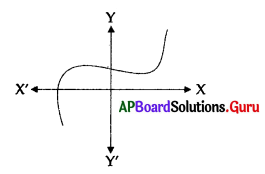A) 0
B) 3
C) 2
D) 1
D) 1

Question 113.
Which of the following graph represents a straight line ?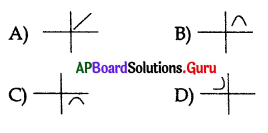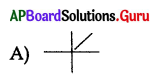Question 114.
How many zeroes are there for the polynomial from the given graph ?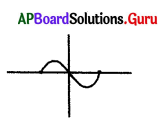A) 4
B) 3
C) 1
D) 2
B) 3

Question 115.
The graph of y = x2 may be ………………….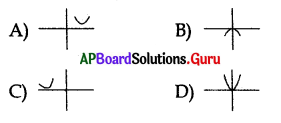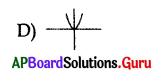Question 116.
If the polynomial has no real zeroes then the graph can be represented as ………………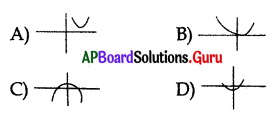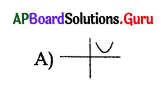Question 117.
-x2 = y can be represented as …………………..